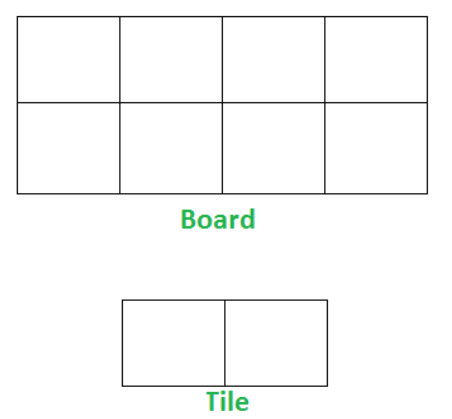Problem title
Difficulty
Avg time to solve

BST queries
Easy
10 mins
Count Distinct Substrings
Moderate
10 mins
Fahrenheit to Celsius
Easy
15 mins
Distinct Subsequences
Moderate
10 mins
Reverse Level Order Traversal
Moderate
30 mins
Max Product Count
Moderate
35 mins
LRU Cache Implementation
Moderate
25 mins
Moderate
30 mins
Tiling Problem
Hard
45 mins
Cycle Detection in a Singly Linked List
Moderate
15 mins21

# Tiling Problem

Difficulty: HARD
Avg. time to solve
45 min

Problem Statement

#### You have been given a board where there are '2' rows and 'N' columns. You have an infinite supply of 2x1 tiles, and you can place a tile in the following ways:

``````1. Horizontally as 1x2 tile
2. Vertically as 2x1 tile
``````

#### Count the number of ways to tile the given board using the available tiles.

##### Note :
``````The number of ways might be large so output your answer modulo 10^9 + 7.
``````

#### Here an example of tile and board for 'N' = 4 :##### Input format :
``````The first and only line of each test case contains an Integer 'N' which denotes the size of the board, i.e. '2' rows and 'N' columns.
``````
##### Output format :
``````For each test case, print the number of ways to tile the board modulo 10^9 + 7.
``````
##### Note:
``````You are not required to print the output explicitly, it has already been taken care of. Just implement the function.
``````
##### Constraints :
``````1 <= N <= 10^18

Where 'N' is the number of columns in the board.

Time limit: 1 sec
``````
##### Sample Input 1 :
``````3
``````
##### Sample Output 1 :
``````3
``````
##### Explanation to Sample Input 1 :
``````For a 2*3 board, there are three ways:
1. Place all 3 tiles vertically.
2. Place first tile vertically and remaining 2 tiles horizontally.
3. Place first 2 tiles horizontally and remaining tiles vertically.
``````
##### Sample Input 2 :
``````4
``````
##### Sample Output 2 :
``````5
``````
##### Explanation to Sample Input 2 :
``````For a 2*4 board, there are five ways:
1. All 4 vertical
2. All 4 horizontal
3. First 2 vertical, remaining 2 horizontal
4. First 2 horizontal, remaining 2 vertical
5. Corner 2 vertical, middle 2 horizontal
``````Console